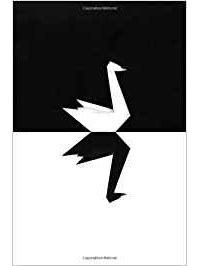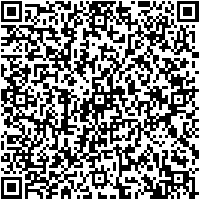Развитие через преодоление конфликтов

### Сибирский Центр медиации

Развитие через преодоление конфликтов

## Black Swan in MathThe extraordinary beauty and conciseness of the formulation of Fermat's great theorem make us look for its visual solution. Let's try to consider Fermat's theorem from the eyes of physicist and geometry. It was from these positions that Pierre de Fermat could find a solution whose main ideas would fit schematically in the fairly wide margins of the book, in a few drawings. However, the paradigms that have prevailed for centuries in mathematics have provided a strong obstacle to finding a solution in the direction that, in modern language, is effective. Skeptics continue to believe that Pierre de Fermat was probably mistaken. Meanwhile, consistent application of the basic principles of physics, geometry, and thought experiment make us think differently.

The famous Fermat theorem formulated by Pierre de Fermat (1601-1665) 383 years ago in 1637 States that the following equation has no solution for any three integers other than zero for the case n > 2:

an + bn = cn                (1)

When n = 2, we are dealing with the usual Pythagorean theorem , and there are an infinite number of solutions to the equation in integers - Pythagorean triples formed by Euclid's formulas. examples of  Pythagorean triples are known: (3, 4, 5); (5, 12, 13); (15, 8, 17) since the time of Euclid, a number of ways to generate Pythagorean triples have been found.

Fermat's theorems make us look for a visual solution. Let's try to consider Fermat's theorem from the point of view of physics and geometry. It is in this direction that there are chances to find a solution, the main ideas of which can be schematically placed on a fairly wide margins of the book.

Let us consider the case of positive integers, i.e. natural numbers a, b, c, and then the case of negative numbers. Since the desired a, b, and c must be integers, any hypercube considered below can be represented as a continuous set of layers, each of which is formed on its hypersurface of dimension n-1, by adding another layer of unit thickness h for each of the n coordinates.

It is more convenient to imagine that a hypercube begins with an elementary unit cube 1n (hereinafter referred to as a the primary cube for short), the center of which coincides with the origin of coordinates. This is the first vertex, then the edges (faces) are increased for each of the n coordinates and the next vertex 1n, as a result, layer № 2 is formed, the edges (faces) and the next vertex of layer № 3 are increased again, and so on. a Hypercube is a set of points in space obtained by combining the unit cube 1n and layers 1 to i. Let us consider the equivalent definitions of a hypercube in the space of integers In (analogy of real coordinate space of n dimensions, Rn).

A hypercube is {1n,S1, S2, . . .Si} or {2n,S1, S2, . . .Si} (3)

The difference is only in the price of the division of the scale, which means the edge of the hypercube 1n. The first definition is convenient to use for many “center in vertices” figures inscribed in each other hypercubes, the second - in the “center in the center of the hypercube”. Both geometrical figures corresponding to each of the just given sets of points of space are transformed into each other due to reflections from planes perpendicular to each of the n coordinate axes, or by cutting the figure into “hyperquadrants” and scaling. The term hyperquadrant is understood, for example, as the subspace (x1, x2, x3, ... xn), where all x> = 0. In this subspace there is one vertex of the hypercube located on the axis of its diagonal. The total number of such hyperquadrants is equal to the number of vertices of the hypercube 2n. Due to the symmetry of the hypercube, it is sufficient to study only one of them, defined as a subset of the non-negative values ​​of xi.

# Proof in one string

Fermat’s theorem can be proved in one drawing and in one formula. We write formula (3) in expanded form, it can count the natural number of layers i. It can be a long enough chain, but easy to understand formula:

1n+ V(n){S1,S2 ,S3, S4 . . Sk} = V(n){Sk+l+1,Sk+l+2. . .Sk+l+m}.

Without changing the generality, we can assume that the inequality b > a > 2 (according to (10) and the last paragraph on the first page) in the form of algebraic expression transform (1) to:

cn - bn = an.

Such an equation obviously has no solution in integers due to the conflict of dimensions in the case of n > 2. On the left side of this equation there are a set of S – hypersurface (n-1) dimension in integer space In (analog of Rn). In contrary on the right side – hypercube n dimension. On the left side one can see the figure corresponding to expression has an irreparable defect of symmetry. On the right side - symmetric figure. That is enough evidence to to conclude that there are no such numeric a, b, c that will help to restore the broken symmetry of the hypercubes as a necessary condition for (1).

From these positions, the Great Fermat Theorem are being proved by careful consideration just one look as in the ancient Indian treatises on mathematics, where the proof in one picture was accompanied by only one word: Look!

### Conclusions

You can significantly simplify the proof of a mathematical theorem and reduce the complexity of the desired solution by applying a number of physical principles:

• sotropy of space;
• Identification of repeatable structures and symmetry properties;
• Mechanical analogy and heuristic search;
• Application of principle of similarity of objects;
• Choosing the appropriate coordinate system and shape of shapes that are appropriate for the objects under study;
• Thought experiment.

Skeptics continue to believe that Pierre de Fermat was probably mistaken. Meanwhile, the consistent application of the basic principles of physics and geometry makes us think differently. Work on Fermat's theorem gave impetus to the development of number theory, which also describes the laws of the physical world, but indirectly. Changing the paradigm allows you to see this relationship in a visual representation.

### References and other sources

1. V. Serpinsky. Pythagorean triangles. - Moscow: Uchpedgiz, 1959. - 111 p.
2. D. E. Joyce. Euclid''s Elements. - Clark University, June 1997. - C. Book X, Proposition XXIX.
3. Douglas W. Mitchell. An Alternative Characterization of All Primitive PythagoreanTriple s // The Mathematical Gazette. - July 2001. - Vol. 85, vol. 503. - Pp. 273-5.
4. Wales''s proof of Fermat's Last Theorem an href. https://en.wikipedia.org/wiki/Wiles
%27s_proof_of_Fermat%27s_Last_Theorem#cite_ref-Singh_1-0 last visit 25.01.2020
5. G. L. Kotkin, V. G. Serbo. Analytical mechanics: Additional questions. Studies'. manual /novosib.UN-T. Novosibirsk, 1987. 84 PP.
6. Buchholz N. N. Basic course of theoretical mechanics. Part 1. 10th ed. — SPb.: LAN, 2009. - 480 p. — ISBN 978-5-8114-0926-6.

* * *

### Добровольные пожертвованияСоюз "Сибирский Центр медиации"  ИНН 5406195342 КПП 860201001 Расч. счёт 40703810967170001448 в ЗАПАДНО-СИБИРСКОЕ ОТДЕЛЕНИЕ№8647 ПАО СБЕРБАНК в городе Тюмени.,  БИК 047102651 Корр. Счёт 30101810800000000651 Назн. платежа: добровольные пожертвования  НДС нет